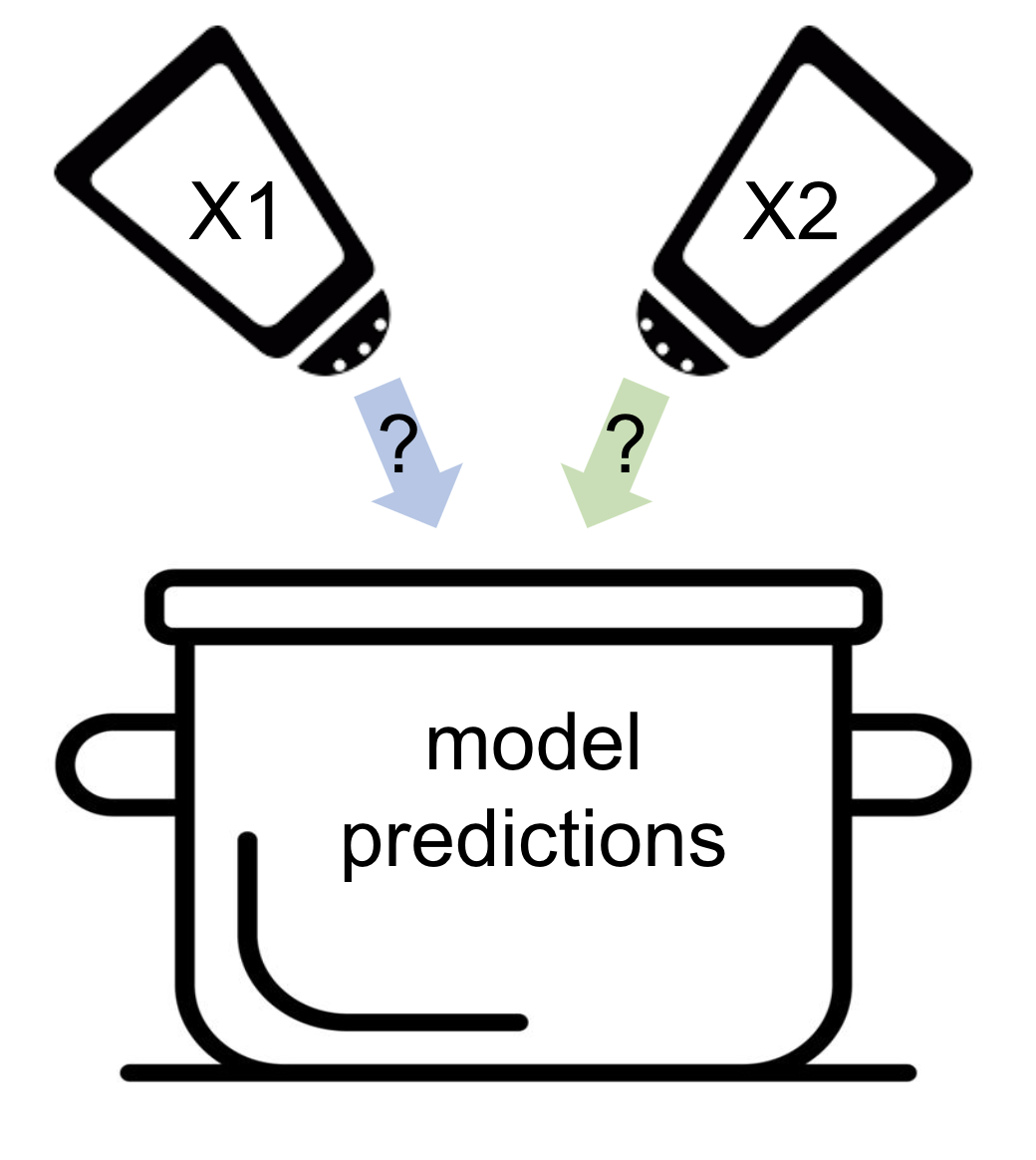## Course Outline

• segmentGetting Started (Don't Skip This Part)
• segmentStatistics and Data Science: A Modeling Approach
• segmentPART I: EXPLORING VARIATION
• segmentChapter 1 - Welcome to Statistics: A Modeling Approach
• segmentChapter 2 - Understanding Data
• segmentChapter 3 - Examining Distributions
• segmentChapter 4 - Explaining Variation
• segmentPART II: MODELING VARIATION
• segmentChapter 5 - A Simple Model
• segmentChapter 6 - Quantifying Error
• segmentChapter 7 - Adding an Explanatory Variable to the Model
• segmentChapter 8 - Models with a Quantitative Explanatory Variable
• segmentPART III: EVALUATING MODELS
• segmentChapter 9 - The Logic of Inference
• segmentChapter 10 - Model Comparison with F
• segmentChapter 11 - Parameter Estimation and Confidence Intervals
• segmentPART IV: MULTIVARIATE MODELS
• segmentChapter 12 - Introduction to Multivariate Models
• segmentChapter 13 - Multivariate Model Comparisons
• segmentFinishing Up (Don't Skip This Part!)
• segmentResources

### list College / Advanced Statistics and Data Science (ABCD)

Book
• High School / Advanced Statistics and Data Science I (ABC)
• High School / Statistics and Data Science I (AB)
• High School / Statistics and Data Science II (XCD)
• College / Statistics and Data Science (ABC)
• College / Advanced Statistics and Data Science (ABCD)

## 12.3 Specifying and Fitting a Multivariate Model

We can see from visualizations of the data that a model that includes both Neighborhood and HomeSizeK might help us make better predictions of PriceK than would a model including only one of these variables. We can write this two-predictor model as a word equation: PriceK= Neighborhood + HomeSizeK + Error. Let’s now see how we would specify and fit such a model.

### Specifying a Multivariate Model in GLM Notation

Building on the notation we used for the one-predictor model, we will specify the two predictor model like this:

$Y_i = b_0+b_1X_{1i}+b_2X_{2i}+e_i$

Although it may look more complicated, on closer examination you can see that it is similar to the single-predictor model in most ways. $$Y_i$$ still represents the outcome variable PriceK, and $$e_i$$, at the end, still represents each data point’s error from the model prediction. And, it still follows the basic structure: DATA = MODEL + ERROR.

Let’s unpack the MODEL part of the equation just a little. Whereas previously we had only one X in the model, we now have two ($$X_{1i}$$ and $$X_{2i}$$). Each X represents a predictor variable. Because it varies across observations it has the subscript i. To distinguish one X from the other, we label one with the subscript 1, the other with 2. The first of these will represent Neighborhood, the second, HomeSizeK, though which X we assign to which variable doesn’t really matter.

Notice, also, that with the additional $$X_{2i}$$ we also add a new coefficient or parameter estimate: $$b_2$$. We said before that the empty model is a one-parameter model because we are estimating only one parameter, $$b_0$$. A single-predictor model (e.g., the home size model) is a two-parameter model: it has both a $$b_0$$ and a $$b_1$$.

This multivariate model is a three-parameter model: $$b_0$$, $$b_1$$, and $$b_2$$.

We can also write this model substituting the variable names for the Xs:

$PriceK_i=b_0+b_1Neighborhood_i+b_2HomeSizeK_i+e_i$

### Fitting a Multivariate Model

Having specified the skeletal structure of the model, we next want to fit the model, which means finding the best fitting parameter estimates (i.e., the values of $$b_0$$, $$b_1$$, and $$b_2$$). By “best fitting” we mean the parameter estimates that reduce error as much as possible around the model predictions.

Although there are several mathematical ways to do this, you can imagine the computer trying every possible combination of three numbers to find the set that results in the lowest Sum of Squares (SS) Error.

It’s a bit like we are cooking up some model predictions and we’ll need to add a little of X1 (HomeSizeK) and a little of X2 (Neighborhood). The best fitting estimates tell us how much of each to add (or subtract) in order to produce the best possible prediction of PriceK.Now enter the lm() code into the window below and run it to get the best fitting parameter estimates for the two-predictor model.

require(coursekata) # delete when coursekata-r updated Smallville <- read.csv("https://docs.google.com/spreadsheets/d/e/2PACX-1vTUey0jLO87REoQRRGJeG43iN1lkds_lmcnke1fuvS7BTb62jLucJ4WeIt7RW4mfRpk8n5iYvNmgf5l/pub?gid=1024959265&single=true&output=csv") Smallville$Neighborhood <- factor(Smallville$Neighborhood) Smallville$HasFireplace <- factor(Smallville$HasFireplace) # use lm() to find the best fitting coefficients # for our multivariate model lm(PriceK~ Neighborhood + HomeSizeK, data = Smallville) # temporary SCT ex() %>% check_error()
CK Code: D1_Code_Specifying_01
Call:
lm(formula = PriceK ~ Neighborhood + HomeSizeK, data = Smallville)

Coefficients:
(Intercept)  NeighborhoodEastside             HomeSizeK
177.25                -66.22                 67.85 

In some ways, this output looks familiar to us. Let’s try to figure out what these parameter estimates mean.

Using the output of lm(PriceK~ Neighborhood + HomeSizeK, data = Smallville), we can write our best fitting model in GLM notation as:

$Y_i = 177.25 + -66.22X_{1i} + 67.85X_{2i}$

As with the single-predictor model, R re-codes Neighborhood, a categorical variable, as a dummy variable and gives it the name NeighborhoodEastside. R codes this dummy variable, represented in the equation as $$X_{1i}$$, as 1 if the house is in Eastside, and 0 if it is not in Eastside.

We also can write the best-fitting model like this, which will help us remember how Neighborhood is dummy coded:

$PriceK_i = 177.25 + -66.22NeighborhoodEastside_{i} + 67.85HomeSizeK_{i}$